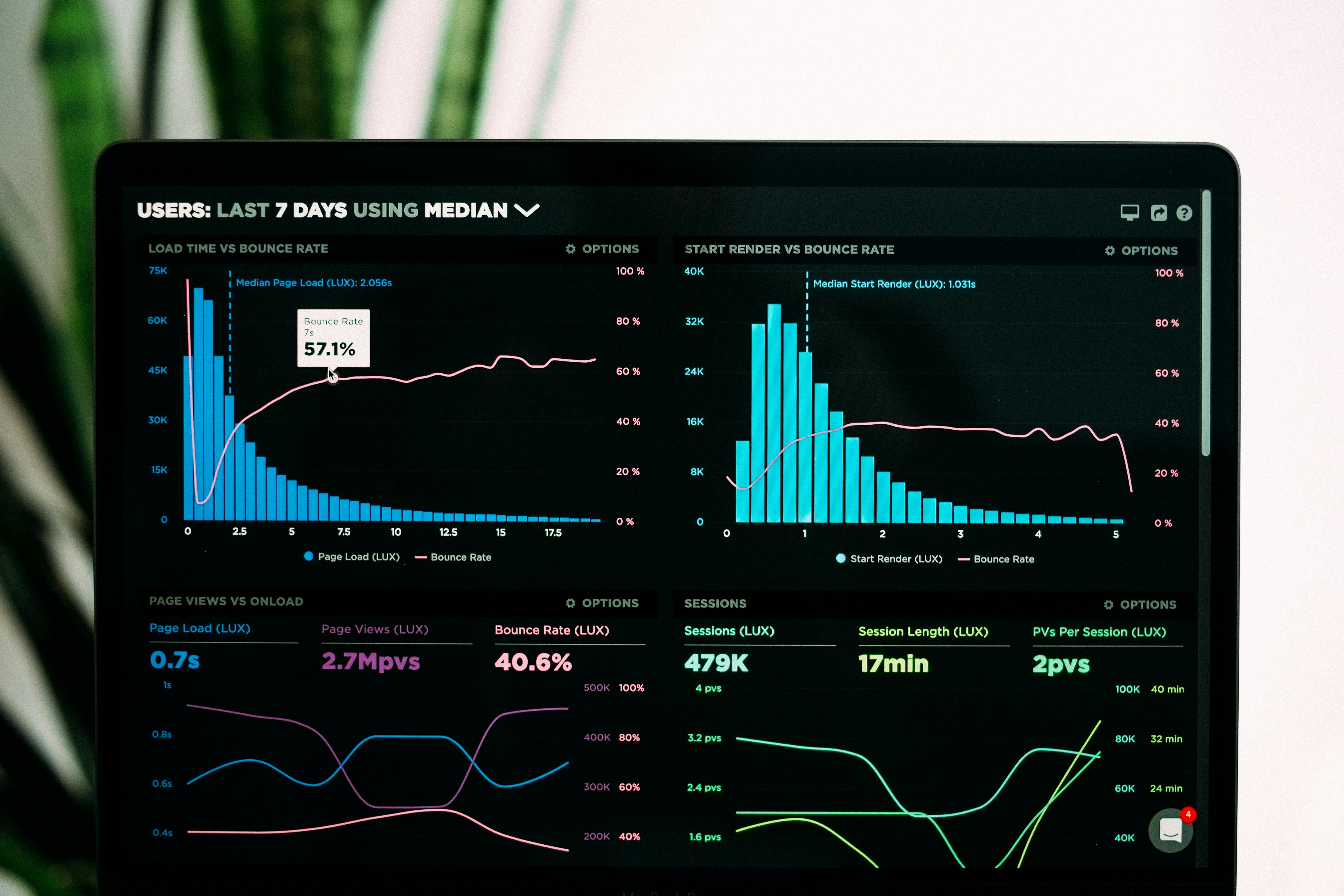Generating random student marks and plotting to graph in Python

# Generating random student marks and plotting to graph in PythonQ. Write a program that does the following

• define a function to generate 1000 integer number randomly between 0 and 100, and save them in a file call it 'data.dat'.
• define a function to read the file contents and count the number of numbers that fall in each range and put the results in a list:
List Name From To
A 90 100
B 80 89
C 70 79
D 60 69
E 50 59
F 0 49
• Use a bar chart to show the list contents.

Solution in python 3

from random import randint
from collections import defaultdict, OrderedDict
import matplotlib.pyplot as plt

# function to generate 1000 integer number randomly between 0 and 100, and save them in a file called 'data.dat'
def generatorZ():
# randint to generate number between
# list comprehension to loop 1000 times
numbers = [randint(0, 101) for i in range(1000)]
# create a data.dat file
with open("data.dat", "w") as f:
# convert our integers to strings
temp = [str(i) for i in numbers]
f.write("\n".join(temp))
# return our array of numbers
return numbers

# function to read the file contents and count the number of numbers that fall in each range and put the results in a list

"A": [90, 101],
"B": [80, 90],
"C": [70, 80],
"D": [60, 70],
"E": [50, 60],
"F": [0, 50],
}
# create an empty integer dictionary such as {'key1':0, 'key2':0, 'key3':0}
groups = defaultdict(int)

# read our earlier created data.dat file
with open("data.dat", "r") as f:
# split lines to array of number string
# convert number string to integers
numbers = [int(i) for i in numbers]
# loop through each numbers
for i in numbers:
# if number falls under a particular group ,example group "A" increment as such groups["A"] +=1
if i in range(value, value):
groups[key] += 1
# continue to next number on finding the correct group, this will save time
# example - if it finds the number 84 fall under group "B" it will not check further if it falls under group "C", "D", "E" & "F"
continue
# sort our dictionary called groups
groups = OrderedDict(sorted(groups.items()))
# return count of each group
return groups

# use our number generator function
numbers = generatorZ()
# use our grades counter function

# using matplotlib to plot our data
fig = plt.figure()
ax.bar(groups,students)
plt.show()


Example output